Elementary theory

A collection of closed formulas of first-order predicate logic. The elementary theory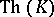of a classof algebraic systems (cf. Algebraic system) of signatureis defined to be the collection of all closed formulas of the first-order predicate logic of signaturethat are true in all systems of. Ifconsists of a single system, then the elementary theory of the classis the elementary theory of the system. Two algebraic systems of the same signature are said to be elementarily equivalent if their elementary theories are the same. An algebraic systemof signatureis called a model of an elementary theoryof signatureif all formulas ofare true in. An elementary theory is called consistent if it has models. A consistent elementary theory is called complete if any two models of it are elementarily equivalent. The class of all models of an elementary theoryis denoted by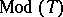. An elementary theoryis called solvable (or decidable) if the set of formulas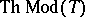(that is, the set of all logical consequences of) is recursive. A classof algebraic systems of signatureis called axiomatizable if there exists an elementary theoryof signaturesuch that. In this caseis called a collection of axioms for. A classis axiomatizable if and only if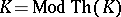. For example, the class of dense linear orders without a smallest or largest element is axiomatizable, its elementary theory is solvable and any two systems of this class are elementarily equivalent, since the elementary theory of this class is complete; moreover, its elementary theory is finitely axiomatizable. The class of finite cyclic groups is not axiomatizable; however, its elementary theory is solvable, and hence recursively axiomatizable. There are examples of finitely-axiomatizable unsolvable elementary theories. They include those of groups, rings, fields, and others. However, a complete recursively-axiomatizable elementary theory is necessarily solvable. Therefore, to prove the solvability of a recursively-axiomatizable elementary theory it is sufficient to observe that it is complete.
Several methods for proving completeness are known. An elementary theory is called categorical in cardinality(cf. Categoricity in cardinality) if all its models of cardinalityare isomorphic. An elementary theory that is categorical in some infinite cardinality and has no finite models is necessarily complete. For example, the elementary theory of algebraically closed fields of a given characteristic is recursively axiomatizable and categorical in every uncountable cardinality; it has no finite models, and therefore it is complete and solvable. In particular, the elementary theory of the field of complex numbers is solvable. Two formulas in the same signature as that of a theoryare equivalent in the theoryif they contain the same variables and if, for any modelofand any assignment of elements ofto their free variables, the formulas are either both true or both false. A complete elementary theoryof finite or countable signature is countably categorical if and only if for everythere are finitely many formulas withfree variables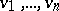such that every formula of the appropriate signature withas free variables is equivalent into one of those formulas. A complete theory of finite or countable signature that is categorical in one uncountable cardinality is also categorical in every other uncountable cardinality. A systemof signatureis called an elementary subsystem of a systemof the same signature ifis a subsystem ofand if for every formula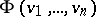of the first-order predicate logic ofwith free variablesand all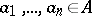, the truth ofinimplies its truth in. An elementary theoryis called model complete if for any two modelsandof it the fact thatis a subsystem ofimplies that it is an elementary subsystem. It turns out that a model-complete theory having a model that can be isomorphically imbedded in every model of the theory is complete. Two systems of the same signature which satisfy the same prenex formulas without existential quantifiers are called universally equivalent. A model-complete elementary theory all models of which are universally equivalent is complete. Using the technique of model completeness one can prove that real-closed fields, in particular, the field of real numbers, have a complete and solvable elementary theory. Among the other solvable elementary theories are those of addition of natural numbers and integers, of Abelian groups, of-adic number fields, of finite fields, of residue class fields, of ordered Abelian groups, and of Boolean algebras.
The general study of unsolvable elementary theories was initiated by A. Tarski in the 1940s, but even earlier, in 1936, A. Church had proved the unsolvability of first-order predicate logic and J. Rosser, also in 1936, had proved the unsolvability of the arithmetic of the natural numbers. The elementary theoryof a classof algebraic systems of the same signatureis said to be inseparable if there is no recursive set of formulas containingand not containing any closed formula which is false in all systems in. The elementary theory of a class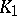of systems of signatureconsisting of a single two-place predicate is called relatively definable in the elementary theory of a class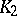of systems of signature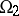if there exist formulas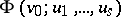and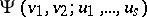ofsuch that for every system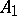inone can find a system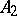inand elements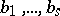infor which the settogether with the predicate, defined onso that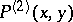is true if and only if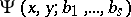is true in, forms an algebraic system isomorphic to. This definition extends naturally to the theory of classesof arbitrary signature. If the elementary theory of a classis inseparable and relatively definable in the elementary theory of a class, then that ofis also inseparable. This makes it possible to prove that the elementary theories of many classes of algebraic systems are inseparable. Here it is convenient to take as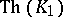the elementary theory of all finite binary relations or that of all finite symmetric relations, or similar elementary theories. Inseparable elementary theories are unsolvable. So are those of the field of rational numbers and of many classes of rings and fields. The unsolvability of the elementary theory of finite groups is an important result of A.I. Mal'tsev.